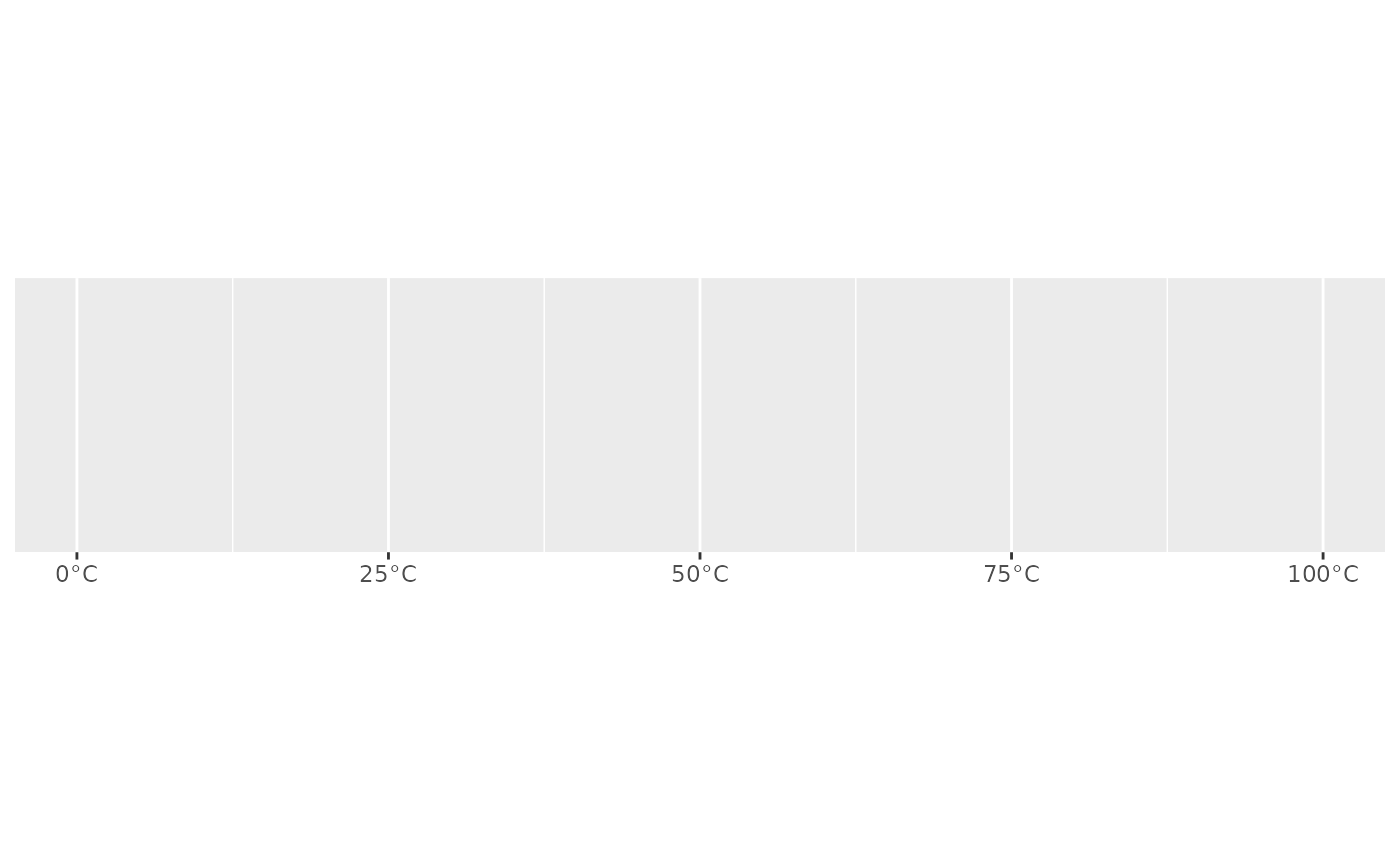Use label_number() force decimal display of numbers (i.e. don't use scientific notation). label_comma() is a special case that inserts a comma every three digits.

## Usage

label_number(
accuracy = NULL,
scale = 1,
prefix = "",
suffix = "",
big.mark = " ",
decimal.mark = ".",
style_positive = c("none", "plus"),
style_negative = c("hyphen", "minus", "parens"),
scale_cut = NULL,
trim = TRUE,
...
)

label_comma(
accuracy = NULL,
scale = 1,
prefix = "",
suffix = "",
big.mark = ",",
decimal.mark = ".",
trim = TRUE,
digits,
...
)

## Arguments

accuracy

A number to round to. Use (e.g.) 0.01 to show 2 decimal places of precision. If NULL, the default, uses a heuristic that should ensure breaks have the minimum number of digits needed to show the difference between adjacent values.

Applied to rescaled data.

scale

A scaling factor: x will be multiplied by scale before formatting. This is useful if the underlying data is very small or very large.

prefix

Additional text to display before the number. The suffix is applied to absolute value before style_positive and style_negative are processed so that prefix = "$" will yield (e.g.) -$1 and (\$1).

suffix

Additional text to display after the number.

big.mark

Character used between every 3 digits to separate thousands.

decimal.mark

The character to be used to indicate the numeric decimal point.

style_positive

A string that determines the style of positive numbers:

• "none" (the default): no change, e.g. 1.

• "plus": preceded by +, e.g. +1.

style_negative

A string that determines the style of negative numbers:

• "hyphen" (the default): preceded by a standard hypen -, e.g. -1.

• "minus", uses a proper Unicode minus symbol. This is a typographical nicety that ensures - aligns with the horizontal bar of the the horizontal bar of +.

• "parens", wrapped in parentheses, e.g. (1).

scale_cut

Named numeric vector that allows you to rescale large (or small) numbers and add a prefix. Built-in helpers include:

• cut_short_scale(): [10^3, 10^6) = K, [10^6, 10^9) = M, [10^9, 10^12) = B, [10^12, Inf) = T.

• cut_long_scale(): [10^3, 10^6) = K, [10^6, 10^12) = M, [10^12, 10^18) = B, [10^18, Inf) = T.

• cut_si(unit): uses standard SI units.

If you supply a vector c(a = 100, b = 1000), absolute values in the range [0, 100) will not be rescaled, absolute values in the range [100, 1000) will be divided by 100 and given the suffix "a", and absolute values in the range [1000, Inf) will be divided by 1000 and given the suffix "b".

trim

Logical, if FALSE, values are right-justified to a common width (see base::format()).

...

Other arguments passed on to base::format().

digitsUse accuracy instead.

## Value

All label_() functions return a "labelling" function, i.e. a function that takes a vector x and returns a character vector of length(x) giving a label for each input value.

Labelling functions are designed to be used with the labels argument of ggplot2 scales. The examples demonstrate their use with x scales, but they work similarly for all scales, including those that generate legends rather than axes.

## Examples

demo_continuous(c(-1e6, 1e6))
#> scale_x_continuous()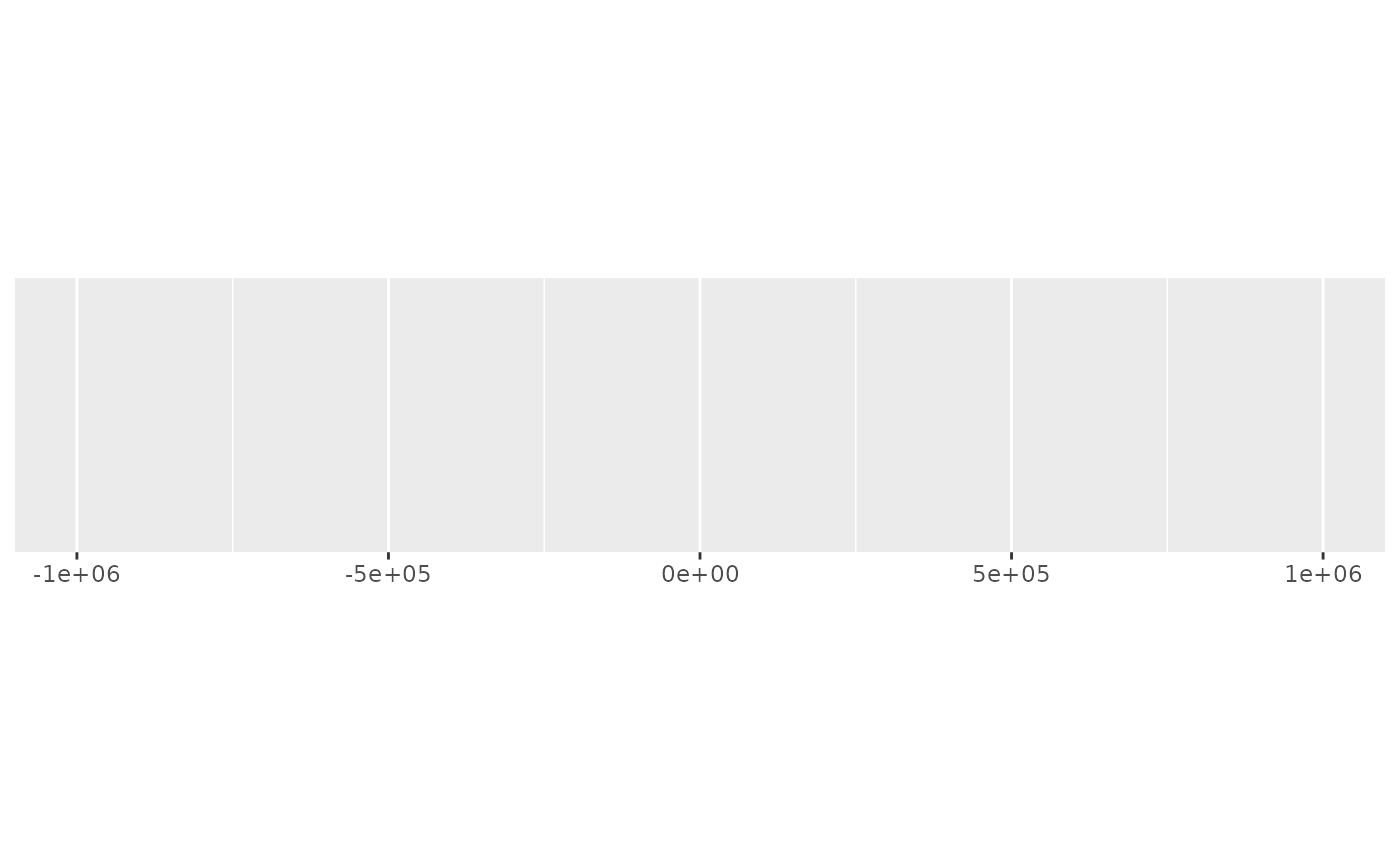demo_continuous(c(-1e6, 1e6), labels = label_number())
#> scale_x_continuous(labels = label_number())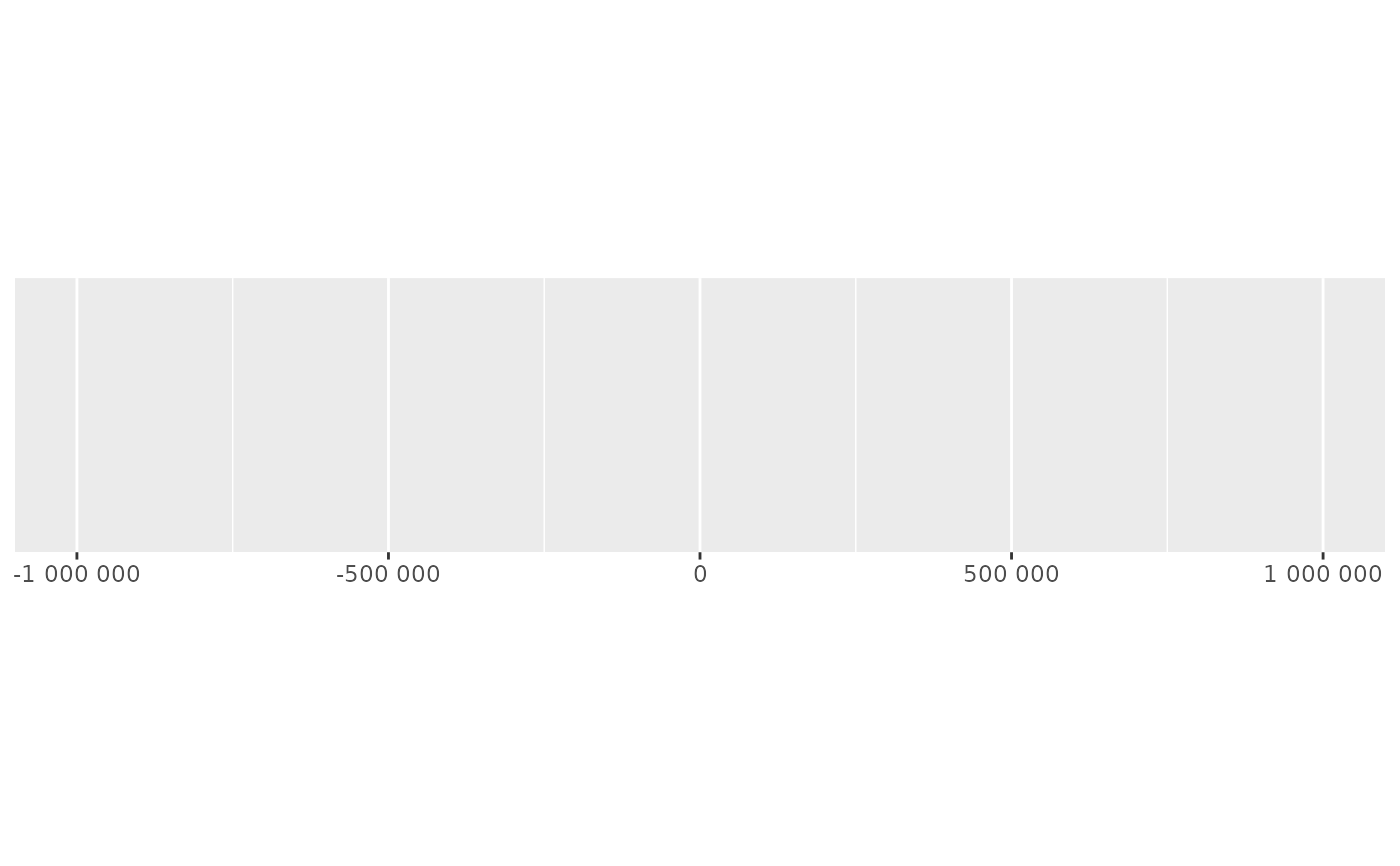demo_continuous(c(-1e6, 1e6), labels = label_comma())
#> scale_x_continuous(labels = label_comma())# Use scale to rescale very small or large numbers to generate
demo_continuous(c(0, 1e6), labels = label_number())
#> scale_x_continuous(labels = label_number())demo_continuous(c(0, 1e6), labels = label_number(scale = 1 / 1e3))
#> scale_x_continuous(labels = label_number(scale = 1/1000))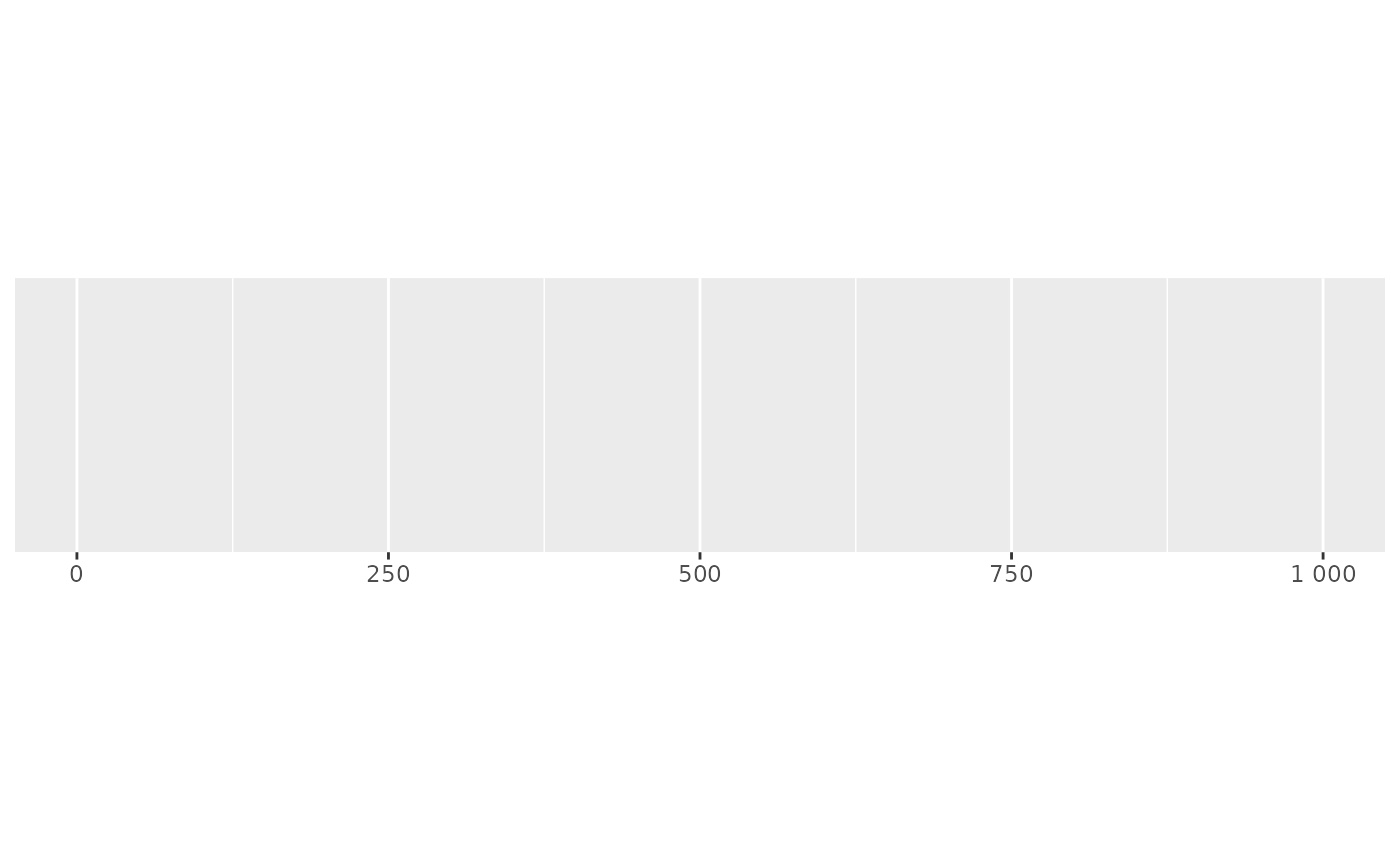demo_continuous(c(0, 1e-6), labels = label_number())
#> scale_x_continuous(labels = label_number())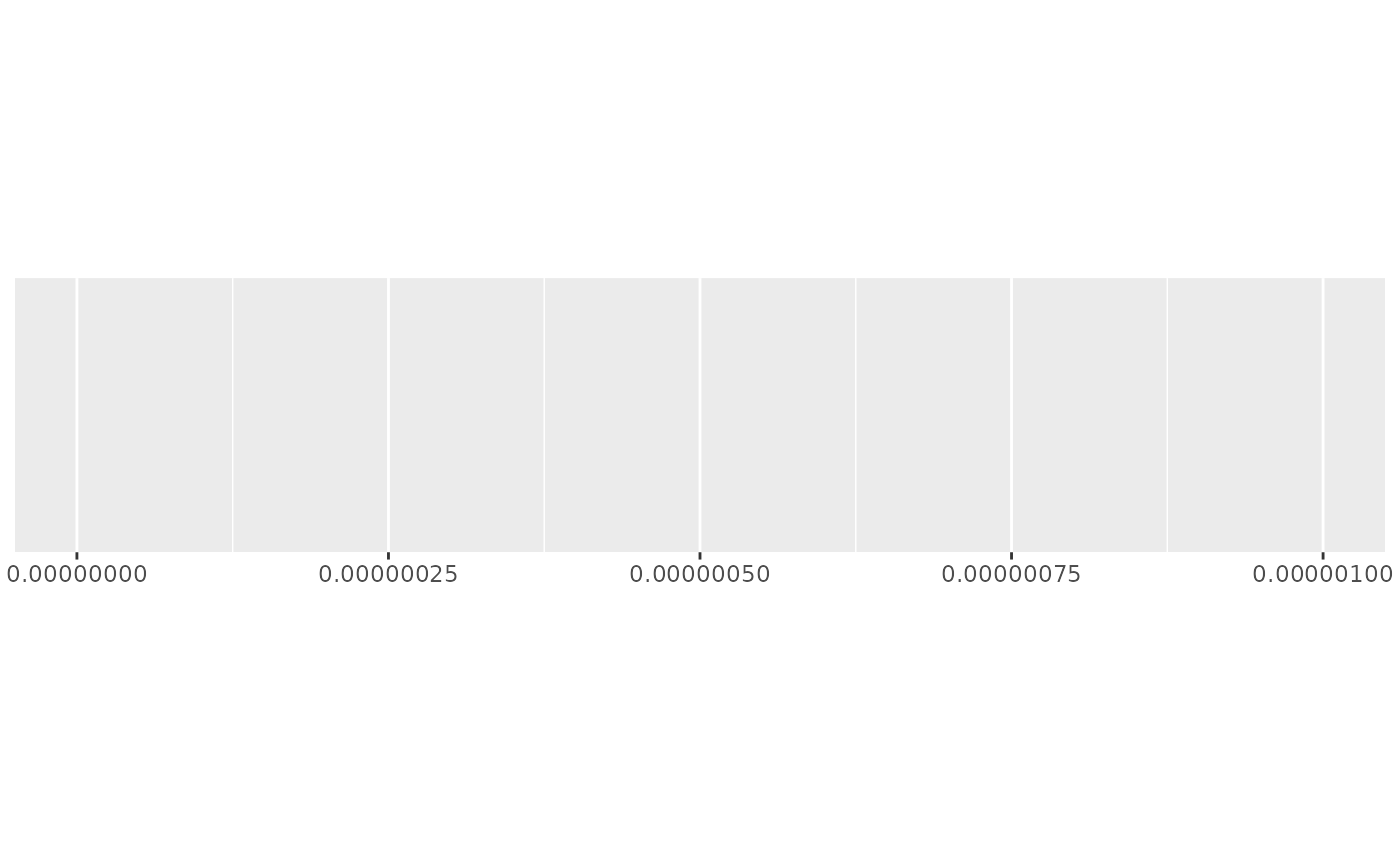demo_continuous(c(0, 1e-6), labels = label_number(scale = 1e6))
#> scale_x_continuous(labels = label_number(scale = 1e+06))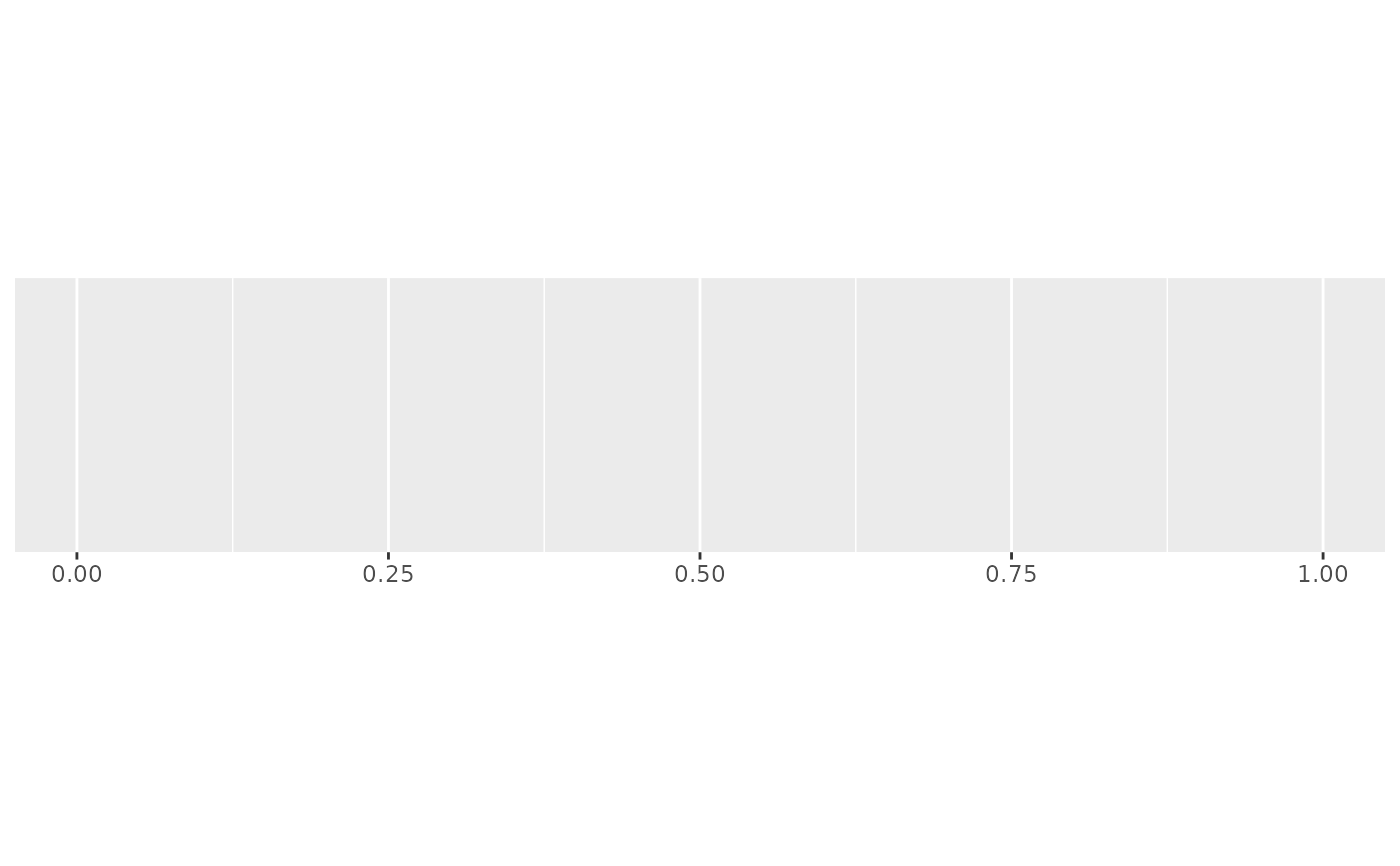#' Use scale_cut to automatically add prefixes for large/small numbers
demo_log10(
c(1, 1e9),
breaks = log_breaks(10),
labels = label_number(scale_cut = cut_short_scale())
)
#> scale_x_log10(breaks = log_breaks(10), labels = label_number(scale_cut = cut_short_scale()))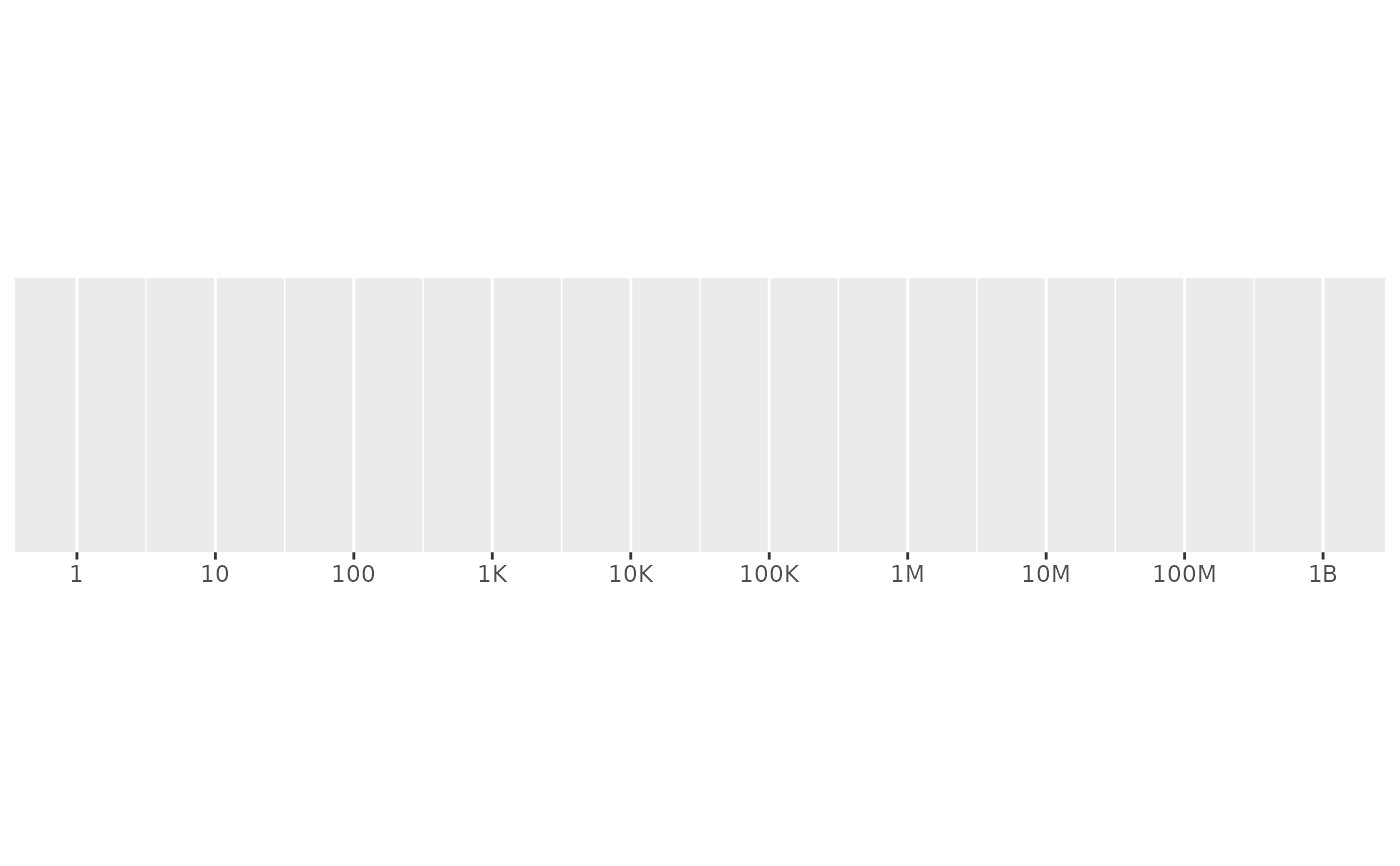demo_log10(
c(1, 1e9),
breaks = log_breaks(10),
labels = label_number(scale_cut = cut_si("m"))
)
#> scale_x_log10(breaks = log_breaks(10), labels = label_number(scale_cut = cut_si("m")))demo_log10(
c(1e-9, 1),
breaks = log_breaks(10),
labels = label_number(scale_cut = cut_si("g"))
)
#> scale_x_log10(breaks = log_breaks(10), labels = label_number(scale_cut = cut_si("g")))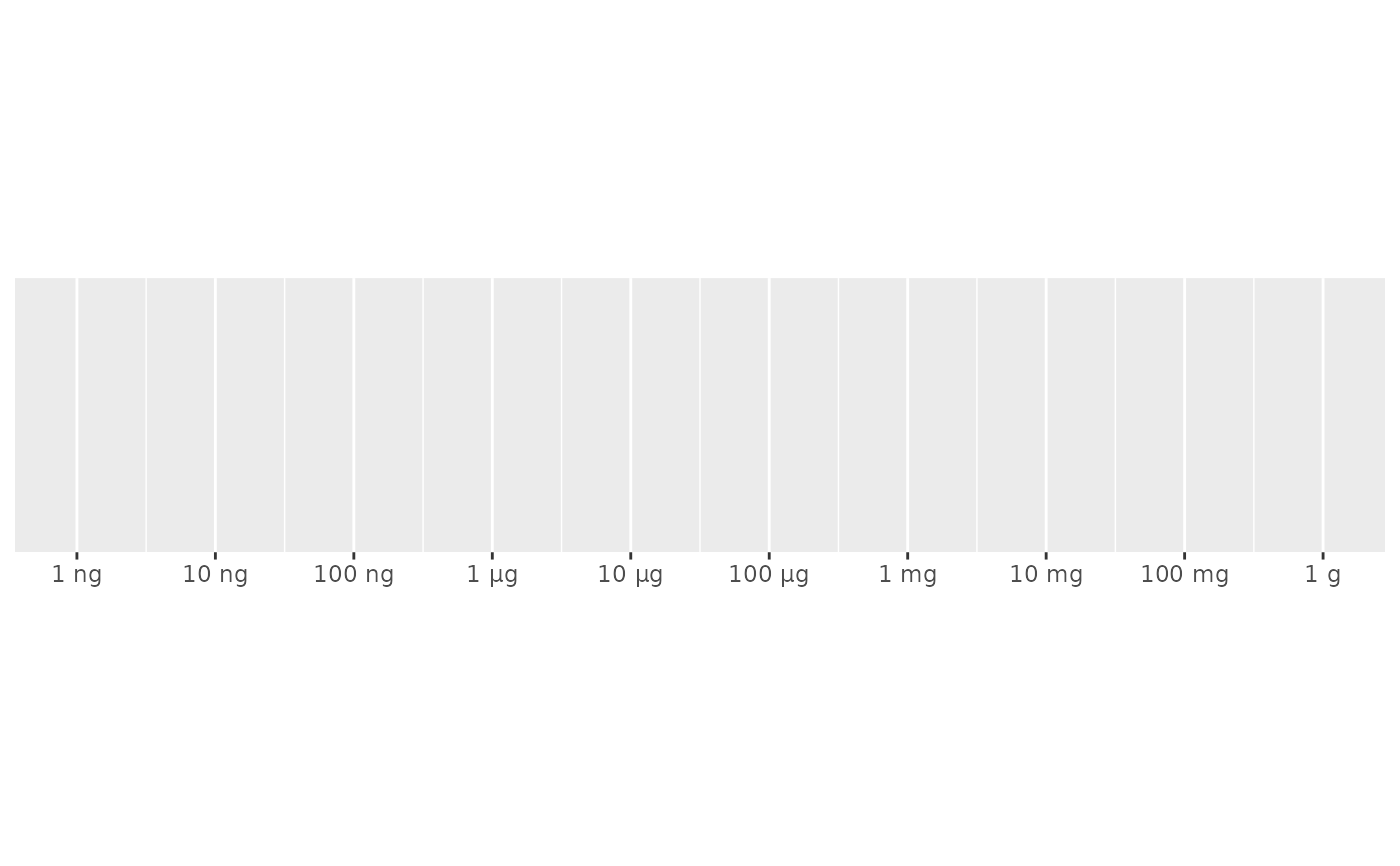# use scale and scale_cut when data already uses SI prefix
# for example, if data was stored in kg
demo_log10(
c(1e-9, 1),
breaks = log_breaks(10),
labels = label_number(scale_cut = cut_si("g"), scale = 1e3)
)
#> scale_x_log10(breaks = log_breaks(10), labels = label_number(scale_cut = cut_si("g"),
#>     scale = 1000))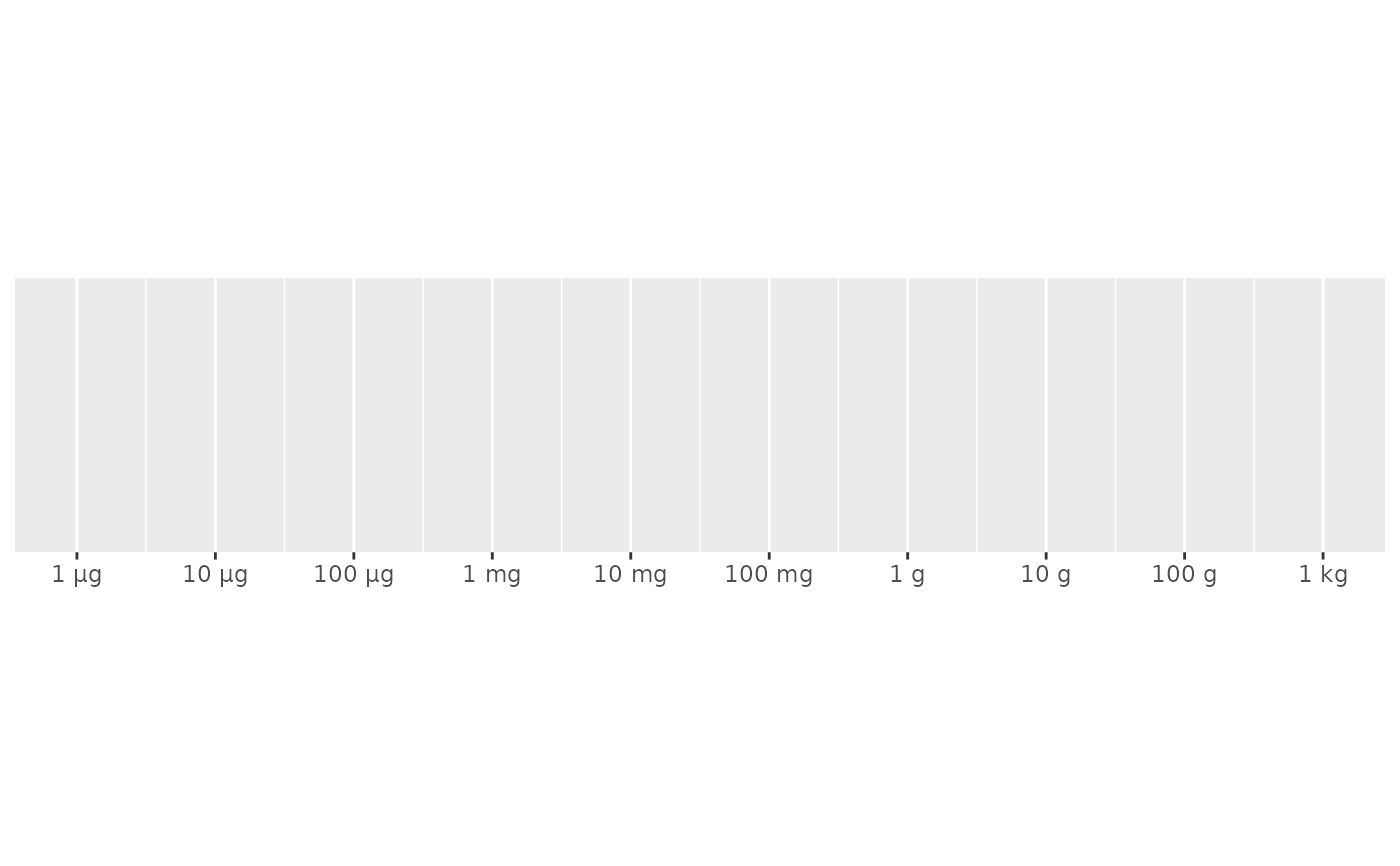#' # Use style arguments to vary the appearance of positive and negative numbers
demo_continuous(c(-1e3, 1e3), labels = label_number(
style_positive = "plus",
style_negative = "minus"
))
#> scale_x_continuous(labels = label_number(style_positive = "plus",
#>     style_negative = "minus"))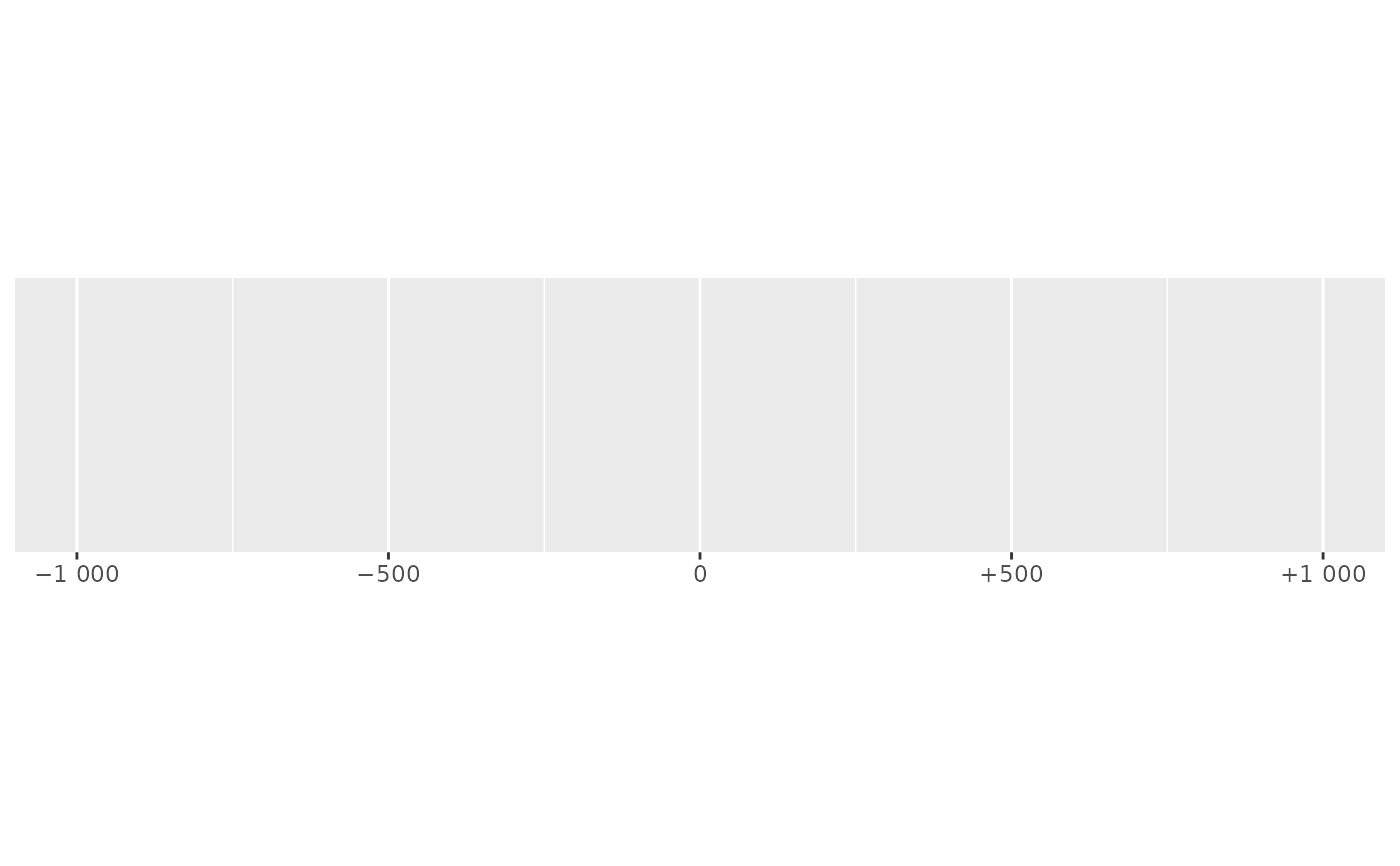demo_continuous(c(-1e3, 1e3), labels = label_number(style_negative = "parens"))
#> scale_x_continuous(labels = label_number(style_negative = "parens"))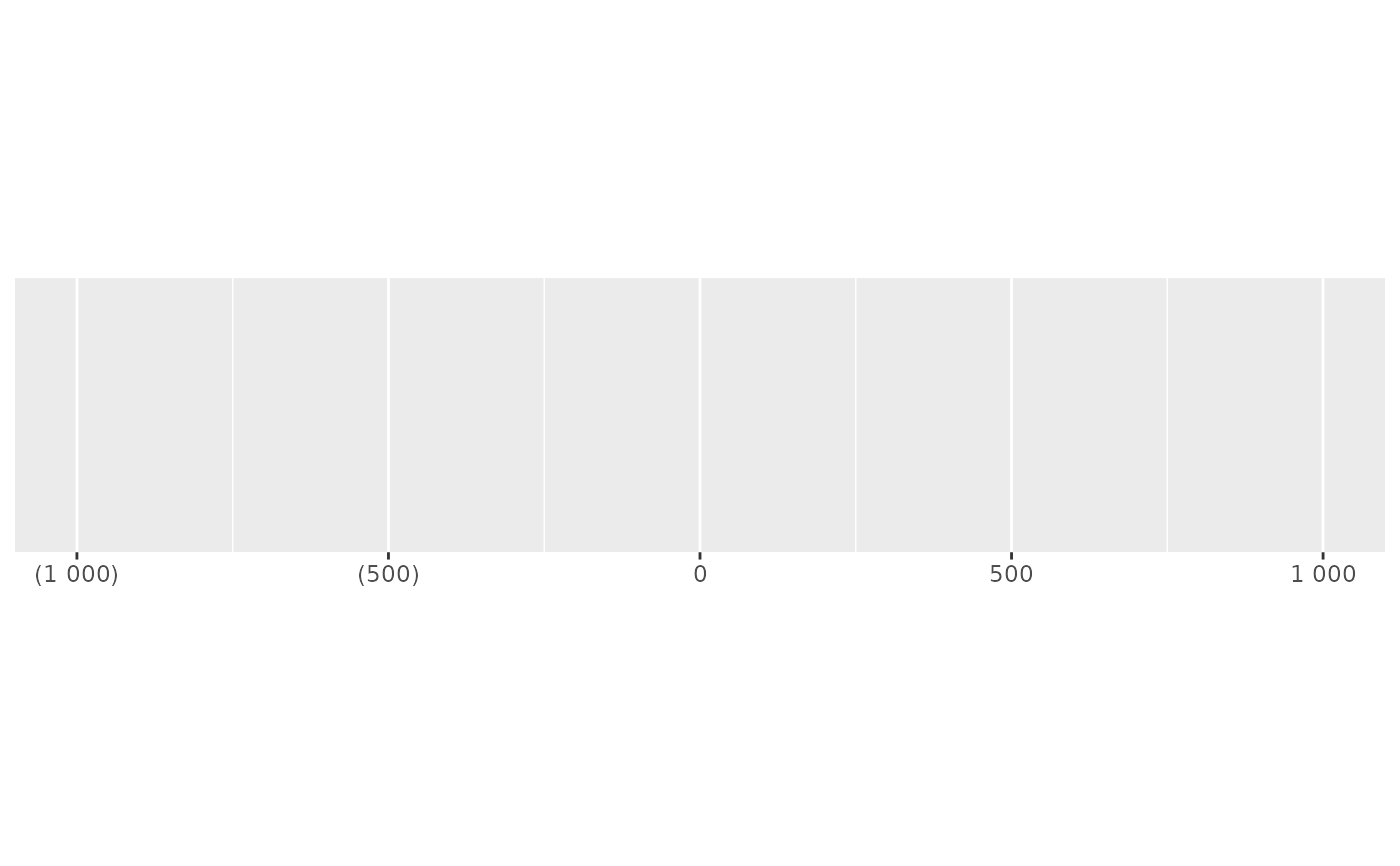# You can use prefix and suffix for other types of display
demo_continuous(c(32, 212), labels = label_number(suffix = "\u00b0F"))
#> scale_x_continuous(labels = label_number(suffix = "°F"))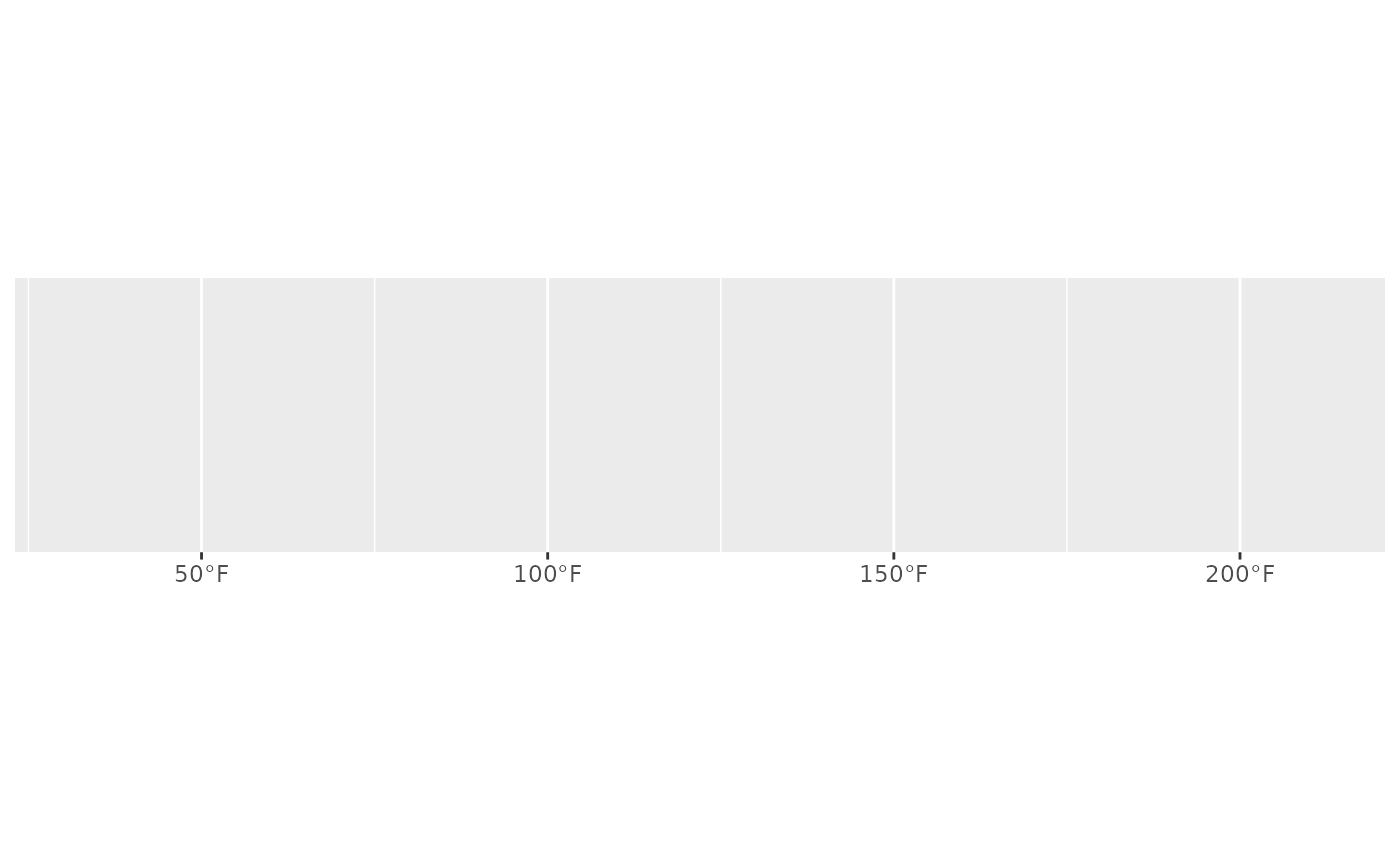demo_continuous(c(0, 100), labels = label_number(suffix = "\u00b0C"))
#> scale_x_continuous(labels = label_number(suffix = "°C"))